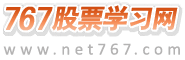767股票学习网 > 股票书籍 > 怎样编写大智慧指标公式 > 正文

# 交易系统

（2）、KDJ交易系统　　　　我们知道如果在D 小于20的区域发生了K线上穿过D线是很好的买入条件；相反,D>80并且好生了D线下穿过了K线,则是很好的卖出条件。如何编辑呢？　　　　第一步：在公式编写栏中分别设定公式的名称、分析周期、参数的各项内容,并编写KD的表达式,并且将K、D表达为两个中间表达式。中间表达式用符号“:=” 　　　　RSB:=(CLOSE-LLV(LOW,N))/(HHV(HIGN,N)-LLV(LOW,N)0*100; K:=SMA(RSV,M1,1); D:=SMA(K,M2,1); 第二步：完成交易系统的结构　　　　ENTERLONG:CROSS(K,D) AND K<20; EXITLONG:CROSS(D,K) AND K>80; 完整表达式：　　　　RSB:=(CLOSE-LLV(LOW,N))/(HHV(HIGN,N)-LLV(LOW,N)0*100; K:=SMA(RSV,M1,1); D:=SMA(K,M2,1); 　　　　ENTERLONG:CROSS(K,D) AND K<20; EXITLONG:CROSS(D,K) AND K>80; 　　　　但是,假设在一个KD强势的市场中,如果股价从下方穿过了30日均线,并且当天的成交量有比较明显的放大,我会买入；我的卖出条件是股价跌下10日均线之下立即抛出。这个交易系统怎么编辑呢？　　买入条件：　　　　KDJ强势：　　　　AA:=”KDJ,D”(9,3,3);（因为KDJ指标中3个参数默认值分别为9、3、3,故我们应在指标中增加默认值）。　　　　A1:=AA>40; 成交量明显放大,量化为大于5日均量的一倍。　　　　A2:=VOL/MA(VOL,5)>2; 股价从下方上穿过了30日均线。　　　　A3:=CROSS(CLOSE,MA(CLOSE,30)); 完整的买入条件为　　　　ENTERLONG:A1 AND A2 AND A3; 卖出条件：　　股价从上方下穿过了5日均线　　A4:=CROSS(MA(CLOSE,5),CLOSE); EXITLONG:A4 AND COUNT(A1 AND A2 AND A3,20)=1;　注意其后的COUNT( )是用来限定卖出信号发生在买入条件发生的20天内。　　那么,完整的公式为：　　AA:=”KDJ,D”(9,3,3); 　　A1:=AA>40; 　　A2:=VOL/MA(VOL,5)>2; 　　A3:=CROSS(CLOSE,MA(CLOSE,30)); 　　A4:=CROSS(MA(CLOSE,5),CLOSE); 　　　　ENTERLONG:A1 AND A2 AND A3; 　　　　EXITLONG:A4 AND COUNT(A1 AND A2 AND A3,20)=1;

 上一篇文章： 五彩K线编辑 下一篇文章： 公式编写实例编写实例

 767股票学习网　|　手机版　|　微信　|　微博　|　联系站长